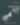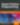# 10 Lodash Features You Can Replace with ES6

JavaScript

Share:

#### Free JavaScript Book!Write powerful, clean and maintainable JavaScript.

RRP \$11.95

Right now, Lodash is the most depended-on npm package, but if you’re using ES6, you might not actually need it.

In this article, we’re going to look at using native collection methods with arrow functions and other new ES6 features to help us cut corners around many popular use cases.

## 1. Map, Filter, Reduce

These collection methods make transforming data a breeze and with near universal support. We can pair them with arrow functions to help us write terse alternatives to the implementations offered by Lodash:

``````_.map([1, 2, 3], function(n) { return n * 3; });
// [3, 6, 9]
_.reduce([1, 2, 3], function(total, n) { return total + n; }, 0);
// 6
_.filter([1, 2, 3], function(n) { return n <= 2; });
// [1, 2]

// becomes

[1, 2, 3].map(n => n * 3);
[1, 2, 3].reduce((total, n) => total + n);
[1, 2, 3].filter(n => n <= 2);
``````

It doesn’t stop here, either. If we’re using a modern browser, we can also use find, some, every and reduceRight too.

Destructuring syntax allows us to get the head and tail of a list without utility functions:

``````_.head([1, 2, 3]);
// 1
_.tail([1, 2, 3]);
// [2, 3]

// becomes

const [head, ...tail] = [1, 2, 3];
``````

It’s also possible to get the initial elements and the last element in a similar way:

``````_.initial([1, 2, 3]);
// -> [1, 2]
_.last([1, 2, 3]);
// 3

// becomes

const [last, ...initial] = [1, 2, 3].reverse();
``````

If you find it annoying that reverse mutates the data structure, then you can use the spread operator to clone the array before calling reverse:

``````const xs = [1, 2, 3];
const [last, ...initial] = [...xs].reverse();
``````

The rest and spread functions allow us to define and invoke functions that accept a variable number of arguments. ES6 introduced dedicated syntaxes for both of these operations:

``````var say = _.rest(function(what, names) {
var last = _.last(names);
var initial = _.initial(names);
var finalSeparator = (_.size(names) > 1 ? ', & ' : '');
return what + ' ' + initial.join(', ') +
finalSeparator + _.last(names);
});

say('hello', 'fred', 'barney', 'pebbles');
// "hello fred, barney, & pebbles"

// becomes

const say = (what, ...names) => {
const [last, ...initial] = names.reverse();
const finalSeparator = (names.length > 1 ? ', &' : '');
return `\${what} \${initial.join(', ')} \${finalSeparator} \${last}`;
};

say('hello', 'fred', 'barney', 'pebbles');
// "hello fred, barney, & pebbles"
``````

## 4. Curry

Without a higher-level language such as [TypeScript] or [Flow], we can’t give our functions type signatures, which makes currying quite difficult. When we receive curried functions, it’s hard to know how many arguments have already been supplied, and which we’ll need to provide next. With arrow functions, we can define curried functions explicitly, making them easier to understand for other programmers:

``````function add(a, b) {
return a + b;
}
// 3

// becomes

const add = a => b => a + b;
// 3
``````

These explicitly curried arrow functions are particularly important for debugging:

``````var lodashAdd = _.curry(function(a, b) {
return a + b;
});
// 0
// function (a, b) {
// /* [wrapped with _.curry & _.partial] */
//   return a + b;
// }

// becomes

const es6Add = a => b => a + b;
// 1
// function b => a + b
``````

If we’re using a functional library like lodash/fp or ramda, we can also use arrows to remove the need for the auto-curry style:

``````_.map(_.prop('name'))(people);

// becomes

people.map(person => person.name);
``````

## 5. Partial

As with currying, we can use arrow functions to make partial application easy and explicit:

``````var greet = function(greeting, name) {
return greeting + ' ' + name;
};

var sayHelloTo = _.partial(greet, 'hello');
sayHelloTo('fred');
// "hello fred"

// becomes

const sayHelloTo = name => greet('hello', name);
sayHelloTo('fred');
// "hello fred"
``````

It’s also possible to use rest parameters with the spread operator to partially apply variadic functions:

``````const sayHelloTo = (name, ...args) => greet('hello', name, ...args);
sayHelloTo('fred', 1, 2, 3);
// "hello fred"
``````

## 6. Operators

Lodash comes with a number of functions that reimplement syntactical operators as functions, so that they can be passed to collection methods.

In most cases, arrow functions make them simple and short enough that we can define them inline instead:

``````_.eq(3, 3);
// true
// 11
_.map([1, 2, 3], function(n) {
return _.multiply(n, 10);
});
// [10, 20, 30]
// 6

// becomes

3 === 3
10 + 1
[1, 2, 3].map(n => n * 10);
[1, 2, 3].reduce((total, n) => total + n);
``````

## 7. Paths

Many of Lodash’s functions take paths as strings or arrays. We can use arrow functions to create more reusable paths instead:

``````var object = { 'a': [{ 'b': { 'c': 3 } }, 4] };

_.at(object, ['a.b.c', 'a']);
// [3, 4]
_.at(['a', 'b', 'c'], 0, 2);
// ['a', 'c']

// becomes

[
obj => obj.a.b.c,
obj => obj.a
].map(path => path(object));

[
arr => arr,
arr => arr
].map(path => path(['a', 'b', 'c']));
``````

Because these paths are “just functions”, we can compose them too:

``````const getFirstPerson = people => people;
const getPostCode = person => person.address.postcode;
const getFirstPostCode = people => getPostCode(getFirstPerson(people));
``````

We can even make higher-order paths that accept parameters:

``````const getFirstNPeople = n => people => people.slice(0, n);

const getFirst5People = getFirstNPeople(5);
const getFirst5PostCodes = people => getFirst5People(people).map(getPostCode);
``````

## 8. Pick

The pick utility allows us to select the properties we want from a target object. We can achieve the same results using destructuring and shorthand object literals:

``````var object = { 'a': 1, 'b': '2', 'c': 3 };

return _.pick(object, ['a', 'c']);
// { a: 1, c: 3 }

// becomes

const { a, c } = { a: 1, b: 2, c: 3 };

return { a, c };
``````

## 9. Constant, Identity, Noop

Lodash provides some utilities for creating simple functions with a specific behavior:

``````_.constant({ 'a': 1 })();
// { a: 1 }
_.identity({ user: 'fred' });
// { user: 'fred' }
_.noop();
// undefined
``````

We can define all of these functions inline using arrows:

``````const constant = x => () => x;
const identity = x => x;
const noop = () => undefined;
``````

Or we could rewrite the example above as follows:

``````(() => ({ a: 1 }))();
// { a: 1 }
(x => x)({ user: 'fred' });
// { user: 'fred' }
(() => undefined)();
// undefined
``````

## 10. Chaining & Flow

Lodash provides some functions for helping us write chained statements. In many cases, the built-in collection methods return an array instance that can be directly chained, but in some cases where the method mutates the collection, this isn’t possible.

However, we can define the same transformations as an array of arrow functions:

``````_([1, 2, 3])
.tap(function(array) {
// Mutate input array.
array.pop();
})
.reverse()
.value();
// [2, 1]

// becomes

const pipeline = [
array => { array.pop(); return array; },
array => array.reverse()
];

pipeline.reduce((xs, f) => f(xs), [1, 2, 3]);
``````

This way, we don’t even have to think about the difference between tap and thru. Wrapping this reduction in a utility function makes a great general purpose tool:

``````const pipe = functions => data => {
return functions.reduce(
(value, func) => func(value),
data
);
};

const pipeline = pipe([
x => x * 2,
x => x / 3,
x => x > 5,
b => !b
]);

pipeline(5);
// true
pipeline(20);
// false
``````

## Conclusion

Lodash is still a great library, and this article only offers a fresh perspective on how the evolved version of JavaScript is allowing us to solve some problems in situations where we would have previously relied on utility modules.

Don’t disregard it. Instead, next time you reach for an abstraction, think about whether a simple function would do instead!

This article was peer reviewed by Mark Brown. Thanks to all of SitePoint’s peer reviewers for making SitePoint content the best it can be!### Dan Prince

Digital Nomad and co-founder of UK based startup Astral Dynamics.

#### New books out now!Learn how Git works, and how to use it to streamline your workflow!Google, Netflix and ILM are Python users. Maybe you should too?